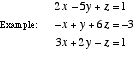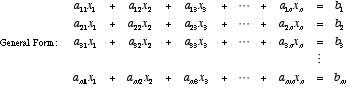index: click on a letter A B C D E F G H I J K L M N O P Q R S T U V W X Y Z A to Z index index: subject areas numbers & symbols sets, logic, proofs geometry algebra trigonometry advanced algebra & pre-calculus calculus advanced topics probability & statistics real world applications multimedia entrieswww.mathwords.com about mathwords website feedback

 Linear System of Equations A system of equations in which each equation is linear. For any linear system, exactly one of the following will be true: There is only one solution, there are infinitely many solutions (consistent), or there are no solutions (inconsistent). A linear system with more equations than variables is called overdetermined, and a linear system with more variables than equations is called underdetermined.See also

 this page updated 19-jul-17 Mathwords: Terms and Formulas from Algebra I to Calculus written, illustrated, and webmastered by Bruce Simmons Copyright © 2000 by Bruce Simmons All rights reserved